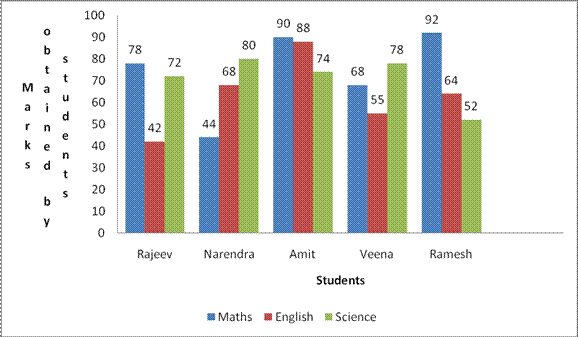## Aptitude Questions and Answers with Solutions

Directions(1-5): Study the following graph carefully and answer the questions given below:
Profit earned by Three companies over the years (Rs. in crores)1.What was the average profit earned by all the three companies in the year 2008 ?
a)Rs.300 crore
b)Rs.400 crore
c)Rs.350 crore
d)Rs.520 crore
e)None of these

2.In which of the following years was the difference between the profits earned by company B and company A the minimum ?
a)2003
b)2004
c)2005
d)2008
e)None of these

3.In which of the following years was the total profit earned by all three companies together the highest ?
a)2004
b)2007
c)2008
d)2009
e)None of these

4.What was the approximate percentage increase in the profit earned by company A from 2006 to 2007 ?
a)36%
b)24%
c)40%
d)20%
e)54%

5.What was the difference between the profit earned by company A in 2004 and the profit earned by company C in 2009 ?
a)Rs.50 crore
b)Rs.1 crore
c)Rs.100 crore
d)Rs.200 crore
e)None of these

Directions(6-10): Read the following bar diagram carefully to answer the questions given below.
Marks obtained by five students in different subjects – Maths, English, and Science in an examination.6.Which student scored the maximum marks in all the subjects taken together ?
a)Rajeev
b)Narendra
c)Amit
d)Veena
e)Ramesh

7.The difference between the average marks obtained by Narendra and Veena is
a)5
b)3
c)4
d)6
e)7

8.If the maximum marks of each subject are 100, what is the approximate percentage of marks got by Ramesh ?
a)67%
b)66%
c)64%
d)69%
e)72%

9.By what percentage, the marks obtained by Amit in science is less that in Maths ?
a)21%
b)24%
c)22%
d)15%
e)18%

10.The ratio of marks obtained by all students in maths and science is respectively
a)89:91
b)23:25
c)25:23
d)93:89
e)None of these

1.b)
2.e)
3.d)
4.a)
5.c)
6.c)
7.b)
8.d)
9.e)
10.d)

### Aptitude Solution:

1.Average profit earned by three companies in 2008
= (400 + 450 + 350)/3
= Rs.400 crore

2.Required difference in 2007 = Rs.25 crore

3.Total profit earned by three companies:
In 2009 = (400 + 425 + 475) = Rs.1300 crore
In 2008 = (450 + 400 + 350) = Rs.1200 crore

4.Required %increase = [(375 – 275)/275] × 100 = 36% (approx)

5.Required difference = (400 – 300) = Rs.100 crore

6.Marks obtained by
Rajeev = 192
Narendra = 192
Amit = 252
Veena = 201
Ramesh = 208

7.Required difference
= (201/3) – (192/3)
= 9/3
= 3

8.Required% = (208/300) × 100 = 69% (approx)

9.Required% = [(90 – 74)/90] × 100 = 18% (approx)

10.Required ratio = 372 : 356 = 93 : 89